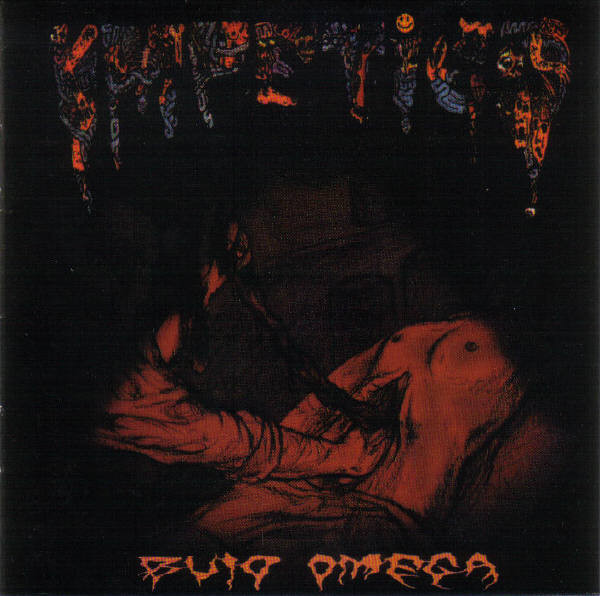## VM-UNDERGROUND

### Extreme Metal Fanzine est. 2012

Filter by: band
[%] - [[0-9]] - [A] - [B] - [C] - [D] - [E] - [F] - [G] - [H] - [I] - [J] - [K] - [L] - [M] - [N] - [O] - [P] - [Q] - [R] - [S] - [T] - [U] - [V] - [W] - [X] - [Y] - [Z]
Filter by: label
[[0-9]] - [A] - [B] - [C] - [D] - [E] - [F] - [G] - [H] - [I] - [J] - [K] - [L] - [M] - [N] - [O] - [P] - [Q] - [R] - [S] - [T] - [U] - [V] - [W] - [X] - [Y] - [Z]
Filter by: style
[B] - [C] - [D] - [E] - [F] - [G] - [H] - [I] - [P] - [S] - [T] - [V]
Filter by: country
[A] - [B] - [C] - [D] - [E] - [F] - [G] - [I] - [L] - [M] - [N] - [P] - [R] - [S] - [T] - [U]
Filter by: vmu-author
[A] - [B] - [C] - [D] - [E] - [F] - [G] - [H] - [I] - [J] - [K] - [L] - [M] - [N] - [O] - [P] - [R] - [S] - [T] - [V] - [X] - [Y] - [Z]

# Impetigo – Buio Omega [EP / Re-Release]## Info

Another EP of Impetigo being re-released on CD, added with the live track of their split EP with Transgressor. Besides that, the CD also has a very cool, extensive CD-rom interactive section with lots of photo’s, concert flyers and much more. Again, a must have for the Impetigo-adepts, so get it!